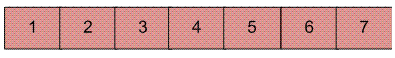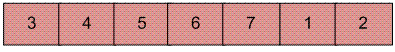# C# Program for Block swap algorithm for array rotation

• Last Updated : 25 May, 2022

Write a function rotate(ar[], d, n) that rotates arr[] of size n by d elements.Rotation of the above array by 2 will make arrayAlgorithm :

```Initialize A = arr[0..d-1] and B = arr[d..n-1]
1) Do following until size of A is equal to size of B

a)  If A is shorter, divide B into Bl and Br such that Br is of same
length as A. Swap A and Br to change ABlBr into BrBlA. Now A
is at its final place, so recur on pieces of B.

b)  If A is longer, divide A into Al and Ar such that Al is of same
length as B Swap Al and B to change AlArB into BArAl. Now B
is at its final place, so recur on pieces of A.

2)  Finally when A and B are of equal size, block swap them.```

Recursive Implementation:

## C#

 `using` `System;` `class` `GFG{``    ` `// Wrapper over the recursive function``// leftRotateRec()``// It left rotates []arr by d.``public` `static` `void` `leftRotate(``int` `[]arr,``                              ``int` `d, ``int` `n)``{``    ``leftRotateRec(arr, 0, d, n);``}` `public` `static` `void` `leftRotateRec(``int` `[]arr, ``int` `i,``                                 ``int` `d, ``int` `n)``{``    ` `    ``// Return If number of elements``    ``// to be rotated is zero or equal``    ``// to array size``    ``if``(d == 0 || d == n)``        ``return``;``    ` `    ``// If number of elements to be rotated``    ``// is exactly half of array size``    ``if``(n - d == d)``    ``{``        ``swap(arr, i, n - d + i, d);``        ``return``;``    ``}``    ` `    ``// If A is shorter``    ``if``(d < n - d)``    ``{``        ``swap(arr, i, n - d + i, d);``        ``leftRotateRec(arr, i, d, n - d);    ``    ``}``    ` `    ``// If B is shorter``    ``else``    ``{``        ``swap(arr, i, d, n - d);``        ` `        ``// This is tricky``        ``leftRotateRec(arr, n - d + i,``                       ``2 * d - n, d);``    ``}``}` `// UTILITY FUNCTIONS``// Function to print an array``public` `static` `void` `printArray(``int` `[]arr,``                              ``int` `size)``{``    ``int` `i;``    ``for``(i = 0; i < size; i++)``        ``Console.Write(arr[i] + ``" "``);``        ` `    ``Console.WriteLine();``}` `// This function swaps d elements``// starting at index fi with d elements``// starting at index si``public` `static` `void` `swap(``int` `[]arr, ``int` `fi,``                        ``int` `si, ``int` `d)``{``    ``int` `i, temp;``    ``for``(i = 0; i < d; i++)``    ``{``        ``temp = arr[fi + i];``        ``arr[fi + i] = arr[si + i];``        ``arr[si + i] = temp;``    ``}``}` `// Driver Code``public` `static` `void` `Main(String[] args)``{``    ``int` `[]arr = { 1, 2, 3, 4, 5, 6, 7 };``    ` `    ``leftRotate(arr, 2, 7);``    ``printArray(arr, 7);    ``}``}` `// This code is contributed by amal kumar choubey`

Output:

`3 5 4 6 7 1 2`

Time Complexity: O(N), where N represents the size of the given array.
Auxiliary Space: O(N), due to recursive stack space.

Iterative Implementation:
Here is iterative implementation of the same algorithm. Same utility function swap() is used here.

## C#

 `// C# code for above implementation``static` `void` `leftRotate(``int` `[]arr, ``int` `d, ``int` `n)``{``    ``int` `i, j;``    ``if``(d == 0 || d == n)``        ``return``;``    ``i = d;``    ``j = n - d;``    ``while` `(i != j)``    ``{``        ``if``(i < j) ``/*A is shorter*/``        ``{``            ``swap(arr, d-i, d+j-i, i);``            ``j -= i;``        ``}``        ``else` `/*B is shorter*/``        ``{``            ``swap(arr, d-i, d, j);``            ``i -= j;``        ``}``        ` `    ``}``    ` `    ``/*Finally, block swap A and B*/``    ``swap(arr, d-i, d, i);``}` `// This code is contributed by Rajput-Ji`

Time Complexity: O(N), where N represents the size of the given array.
Auxiliary Space: O(1), no extra space is required, so it is a constant.

Please see following posts for other methods of array rotation:
https://www.geeksforgeeks.org/array-rotation/
https://www.geeksforgeeks.org/program-for-array-rotation-continued-reversal-algorithm/

References:
http://www.cs.bell-labs.com/cm/cs/pearls/s02b.pdf
Please write comments if you find any bug in the above programs/algorithms or want to share any additional information about the block swap algorithm.

Please refer complete article on Block swap algorithm for array rotation for more details!

My Personal Notes arrow_drop_up